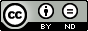### Fuzzy Equivalence Relation, Fuzzy Congrunce Relation and Fuzzy Normal Subgroups on Group G Over T-Norms

Rasul Rasuli

#### Abstract

In this study, by using t-norms, fuzzy equivalence relation, fuzzy congrunce relation on group G, fuzzy relation of subgroup H of group G, fuzzy normal subgroups of fuzzy subgroups, direct product of fuzzy subgroups(normal fuzzy subgroups) are introduced and some the their properties will be discussed. Next by using group homomorphisms, the image and pree image of them will be investigated.

#### Keywords

Fuzzy set theory, norms, groups, congruence relations, homomorphisms, direct

PDF

#### References

M. T. Abu Osman, On some products of fuzzy subgroups, Fuzzy Sets and Systems, 24 (1987), 79-86.

J. M. Anthony and H. Sherwood, Fuzzy groups redefined, J. Math. Anal. Appl., 69(1979), 124-130.

D. R. Bae and J. P. Kim, Fuzzy congruences in groups, Fuzzy Sets and Systems, 85(1997), 115-120.

I. Beg, Fuzzy multivalued functions, Bull. Allahabad Math. Soc., 21(2006), 41-104.

I. Beg and S. Ashraf, Fuzzy similarity and measure of similarity with Lukasiewicz implicator, New Math.

Natural Computation, 4(2008), 191-206.

I. Beg and S. Ashraf, Fuzzy inclusion and fuzzy similarity with Godel implication operator, New Math.

Natural Computation, 5(2009), 617-633.

I. Beg and S. Ashraf, Fuzzy dissimilarity measures and distance functions, Fuzzy Information and Engi

neering, 1(2009), 205-217.

I. Beg and S. Ashraf, Similarity measures for fuzzy sets, Appl. and Comput. Math., 8(2009), 192-202.

I. Beg and S. Ashraf, Fuzzy Relations, Lambert Academic Publisher, Germany, 2009.

V. V. Cross and T. A. Sudkamp, Similarity and Compatibility in Fuzzy Set Theory, Springer-Verlag,

Heidelberg 2002.

D. Dubois and H. Prade, Fundamentals of Fuzzy Sets, Kluwer Academic Publishers, Dordrecht - 2000.

S. Gottwald, Fuzzy Sets and Fuzzy Logic, Vieweg, Wiesbaden, 1993.

U. Hohle and S. E. Rodabaugh, Mathematics of Fuzzy Sets: Logic, Topology, and Measure Theory, The

Hand book of Fuzzy Mathematics Series, 1998.

E. E. Kerre, Introduction to the Basic Principles of Fuzzy Set Theory and some of its Applications, Com

munication and Cognition, 1991.

E. E. Kerre, Basic principles of fuzzy set theory for the representation and manipulation of imprecision

and uncertainty, Communication and Cognition, Gent, second revised edition (1993), 1-158.

E. Kerre, An overview of fuzzy relational calculus and its applications, Lecture Notes in Computer Science,

(2007), 1-13.

I. J. Kumar, P. K. Saxena and P. Yadava, Fuzzy normal subgroups and fuzzy quotients, Fuzzy Sets and

Systems, 46(1992), 121-132.

N. Kuroki, Fuzzy congruences and Fuzzy normal subgroups, Inform. Sci., 60(1992), 247-361.

H. X. Li and V. C. Yen, Fuzzy Sets and Fuzzy Decision-Making, CRC Press. Inc., London, 1995.

D. S. Malik and J. N. Mordeson, A note on fuzzy relations and fuzzy groups, Inform. Sci., 56(1991),

-198.

K. Menger, Probabilistic theories of relations, Proc. Nat. Acad. Sci. USA, 37 (1951), 178-180.

C. V. Negoita and D. A. Ralescu, Representation theorems for fuzzy concepts, Kybernetes, 4(1975), 169-174.

H. T. Nguyen and E. A. Walker, Fuzzy Logic, CRC Press. Boston, 2006.

K. Peeva and Y. Kyosev, Fuzzy Relational Calculus, World Scientific, New Jersey 2004.

H. Poincare, La Science et Hypothese, Flammarion, Paris, 1902.

R. Rasuli, Fuzzy Ideals of Subtraction Semigroups with Respect to A t-norm and A t-conorm, The Journal

of Fuzzy Mathematics Los Angeles, 24 (4) (2016), 881-892.

R. Rasuli, Fuzzy modules over a t-norm, Int. J. Open Problems Compt. Math., 9 (3) (2016), 12-18.

R. Rasuli, Fuzzy Subrings over a t-norm , The Journal of Fuzzy Mathematics Los Angeles, 24 (4) (2016),

-1000.

R. Rasuli, Norms over intuitionistic fuzzy subrings and ideals of a ring, Notes on Intuitionistic Fuzzy Sets,

(5) (2016), 72-83.

R. Rasuli, Norms over fuzzy Lie algebra, Journal of New Theory, 15(2017), 32-38.

R. Rasuli, Fuzzy subgroups on direct product of groups over a t-norm, Journal of Fuzzy Set Valued Analysis,

(2017), 96-101.

R. Rasuli, Characterizations of intuitionistic fuzzy subsemirings of semirings and their homomorphisms by

norms, Journal of New Theory, 18(2017), 39-52.

R. Rasuli, Intuitionistic fuzzy subrings and ideals of a ring under norms, LAP LAMBERT Academic

publishing, 2017, ISBN: 978-620-2-06926-7.

R. Rasuli, Characterization of Q-Fuzzy subrings (Anti Q-Fuzzy Subrings) with respect to a T-norm (TConorms), Journal of Information and Optimization Science, 31(2018), 1-11.

R. Rasuli, T -Fuzzy Submodules of R × M, Journal of New Theory, 22(2018), 92-102.

R. Rasuli, Fuzzy subgroups over a T -norm, Journal of Information and Optimization Science, 39(2018),

-1765.

A. Rosenfeld, Fuzzy Groups, J. Math. Anal. Appl., 35(1971), 512-517.

B. Russell, Vagueness, Australian J. Phil., 1(1923), 84-92.

M. Samhan, Fuzzy congruences on semigroups, Inform. Sci., 74(1993), 165-175.

L. A. Zadeh, Similarity relations and fuzzy orderings, Inf. Sci., 3(1971), 177-200.

L. A. Zadeh, Fuzzy Set Theory and its Applications, Kluwer Academic Publishers, Boston, 1991.

L. A. Zadeh, Fuzzy sets, Inform. Control., 8(1965), 338-353.

L. A. Zadeh, Generalized theory of uncertainty (GTU)principal concepts and ideas, Comput. Statist. Data

Anal., 51(2006), 15-46.

L. A. Zadeh, How do you define similarity?, Private Communication, 2007.

DOI: https://doi.org/10.24203/ajfam.v7i2.5736

### Refbacks

• There are currently no refbacks.

Copyright (c) 2019 Asian Journal of Fuzzy and Applied Mathematics